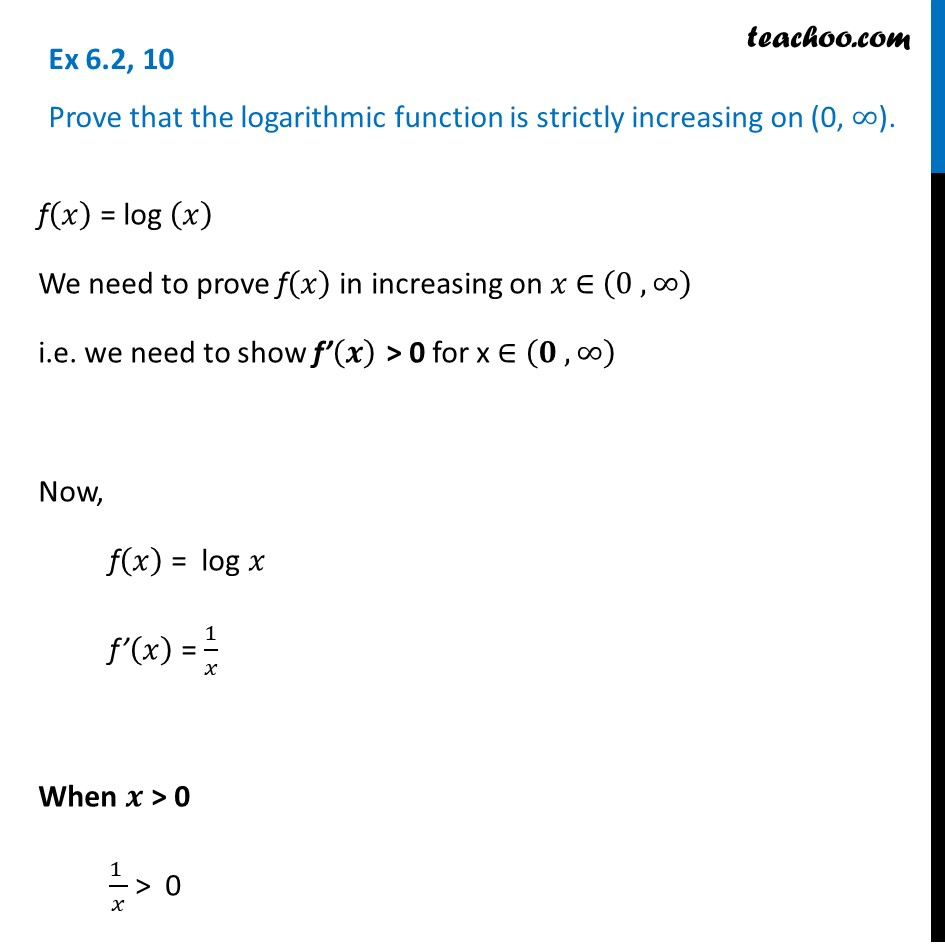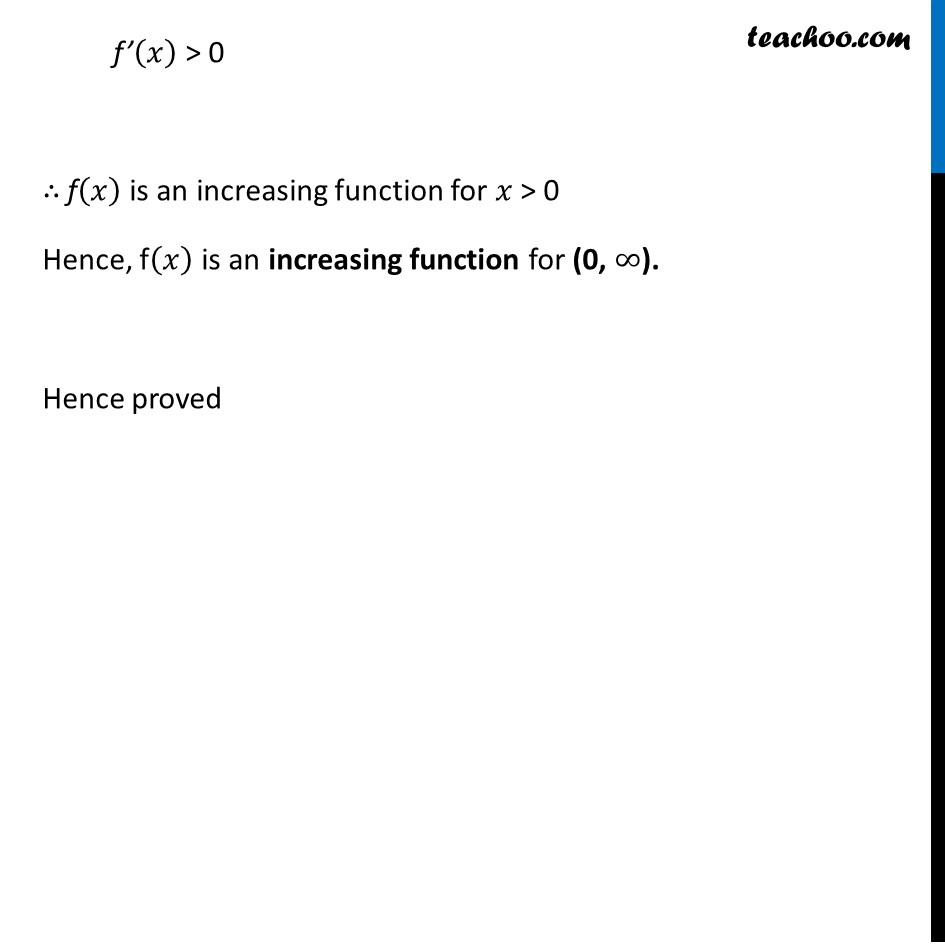Ex 6.2

Chapter 6 Class 12 Application of Derivatives
Serial order wiseLearn in your speed, with individual attention - Teachoo Maths 1-on-1 Class

### Transcript

Ex 6.2, 10 Prove that the logarithmic function is strictly increasing on (0, ∞).f(𝑥) = log (𝑥) We need to prove f(𝑥) in increasing on 𝑥 ∈ (0 , ∞) i.e. we need to show f’(𝒙) > 0 for x ∈ (𝟎 , ∞) Now, f(𝑥) = log 𝑥 f’(𝑥) = 1/𝑥 When 𝒙 > 0 (1 )/𝑥 > 0 f’(𝑥) > 0 ∴ f(𝑥) is an increasing function for 𝑥 > 0 Hence, f(𝑥) is an increasing function for (0, ∞). Hence proved